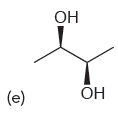# Problem:  Identify whether each of the following compounds is chiral or achiral:

85% (78 ratings)
###### Problem Details

Identify whether each of the following compounds is chiral or achiral:What scientific concept do you need to know in order to solve this problem?

Our tutors have indicated that to solve this problem you will need to apply the Predicting Chirality concept. If you need more Predicting Chirality practice, you can also practice Predicting Chirality practice problems.

What is the difficulty of this problem?

Our tutors rated the difficulty of Identify whether each of the following compounds is chiral ...as low difficulty.

How long does this problem take to solve?

Our expert Organic tutor, Jonathan took 1 minute and 40 seconds to solve this problem. You can follow their steps in the video explanation above.

What textbook is this problem found in?

Our data indicates that this problem or a close variation was asked in Organic Chemistry - Klein 1st Edition. You can also practice Organic Chemistry - Klein 1st Edition practice problems.